American Journal of Modern Physics
Volume 4, Issue 5-1, October 2015, Pages: 52-58

Hyper-Representations by Non Square Matrices Helix-Hopes

T. Vougiouklis, S. Vougiouklis

Democritus University of Thrace, School of Education, Athens, Greece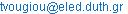(T. Vougiouklis)

T. Vougiouklis, S. Vougiouklis. Hyper-Representations by Non Square Matrices. Helix-Hopes. American Journal of Modern Physics. Special Issue: Issue I: Foundations of Hadronic Mathematics. Vol. 4, No. 5-1, 2015, pp. 52-58. doi: 10.11648/j.ajmp.s.2015040501.17

Abstract: Hyperstructure theory can overcome restrictions which ordinary algebraic structures have. A hyperproduct on non-square ordinary matrices can be defined by using the so called helix-hyperoperations. We define and study the helix-hyperstructures on the representations and we extend our study up to Lie-Santilli theory by using ordinary fields. Therefore the related theory can be faced by defining the hyperproduct on the extended set of non square matrices. The obtained hyperstructure is an Hv-algebra or an Hv-Lie-alebra.

Keywords: Hyperstructures, Hv-Structures, H/V-Structures, Hope, Helix-Hope

Contents

1. Introduction

We deal with the largest class of hyperstructures called Hv-structures introduced in 1990 ,, which satisfy the weak axioms where the non-empty intersection replaces the equality.

Basic definitions:

Definitions 1.1 In a set H equipped with a hyperoperation, which we abbreviate it by hope ×:H´H®P(H), we abbreviate by WASS the weak associativity: (xy)zÇx(yz)¹Æ, "x,y,zÎH and by COW the weak commutativity: xyÇyx¹Æ, "x,yÎH.

The hyperstructure (H,×) is called Hv-semigroup if it is WASS and is called Hv-group if it is reproductive Hv-semigroup: xH=Hx=H, "xÎH. (R,+,×) is called Hv-ring if (+) and (×) are WASS, the reproduction axiom is valid for (+) and (×) is weak distributive with respect to (+):

x(y+z)Ç(xy+xz)¹Æ, (x+y)zÇ(xz+yz)¹Æ, "x,y,zÎR.

For more definitions and applications on Hv-structures, see books ,, and the survey papers ,,. An extreme class is the following : An Hv-structure is very thin iff all hopes are operations except one, with all hyperproducts singletons except only one, which is a subset of cardinality more than one. Therefore, in a very thin Hv-structure in a set H there exists a hope (×) and a pair (a,b)ÎH2 for which ab=A, with cardA>1, and all the other products, with respect to any other hopes (so they are operations), are singletons.

The fundamental relations β* and γ* are defined, in Hv-groups and Hv-rings, respectively, as the smallest equivalences so that the quotient would be group and ring, respectively ,,,,,. The way to find the fundamental classes is given by analogous theorems to the following:

Theorem 1.2 Let (H,×) be an Hv-group and let us denote by U the set of all finite products of elements of H. We define the relation β in H as follows: xβy iff {x,y}Ìu where uÎU. Then the fundamental relation β* is the transitive closure of the relation β.

The main point of the proof of this theorem is that β guaranties that the following is valid: Take two elements x,y such that {x,y}ÌuÎU and any hyperproduct where one of these elements is used. Then, if this element is replaced by the other, the new hyperproduct is inside the same fundamental class where the first hyperproduct is. Therefore, if the ‘hyperproducts’of the above β-classes are ‘products’, then, they are fundamental classes. Analogously for the γ in Hv-rings.

An element is single if its fundamental class is a singleton.

Motivation for Hv-structures:

We know that the quotient of a group with respect to an invariant subgroup is a group.

Marty states that, the quotient of a group with respect to any subgroup is a hypergroup.

Now, the quotient of a group with respect to any partition is an Hv-group.

Definition 1.3 Let (H,×), (H,Ä) be Hv-semigroups defined on the same set H. (×) is smaller than (Ä), and (Ä) greater than (×), iff there exists automorphism

fÎAut(H,Ä) such that xyÌf(xÄy), "xÎH.

Then (H,Ä) contains (H,×) and write ×£Ä. If (H,×) is structure, then it is basic and (H,Ä) is an Hb-structure.

The Little Theorem . Greater hopes of the ones which are WASS or COW, are also WASS and COW, respectively.

The fundamental relations are used for general definitions of hyperstructures. Thus, to define the general Hv-field one uses the fundamental relation γ*:

Definition 1.4 ,,. The Hv-ring (R,+,×) is called Hv-field if the quotient R/γ* is a field.

Let ω* be the kernel of the canonical map from R to R/γ*; then we call reproductive Hv-field any Hv-field (R,+,×) if the following axiom is valid:

x(R-ω*) = (R-ω*)x = R-ω*, "xÎR-ω*.

From the above a new class is introduced ,:

Definition 1.5 The Hv-semigroup (H,×) is called h/v-group if the H/β* is a group.

Similarly the h/v-rings, h/v-fields, h/v-modulus, h/v-vector spaces etc, are defined. The h/v-group is a generalization of the Hv-group since the reproductivity is not necessarily valid. Sometimes a kind of reproductivity of classes is valid, i.e. if H is partitioned into equivalence classes σ(x), then the quotient is reproductive xσ(y)=σ(xy)=σ(x)y, "xÎH .

An Hv-group is cyclic ,, if there is element, called generator, which the powers have union the underline set, the minimal power with this property is the period of the generator. If there exists an element and a special power, the minimum one, is the underline set, then the Hv-group is called single-power cyclic.

To compare classes we can see on small sets. The problem of enumeration and classification of Hv-structures, or of classes of them, is complicate in Hv-structures because we have great numbers. The partial order in Hv-structures, introduced in , restrict the problem in finding the minimal Hv-structures, up to isomorphism. We have results recently by Bayon & Lygeros as the following ,:

In sets with three elements: Up to isomorphism, there are 6.494 minimal Hv-groups. The 137 are abelians; the 6.152 are cyclic. The number of Hv-groups with three elements, up to isomorphism, is 1.026.462. The 7.926 are abelians; 1.013.598 are cyclic. 16 are very thin. Abelian Hv-groups with 4 elements are, 8.028.299.905, the 7.995.884.377.

Definitions 1.6 ,, Let (R,+,×) be Hv-ring, (M,+) be COW Hv-group and there exists an external hope:

R´M®P(M): (a,x)®ax,

such that, "a,bÎR and "x,yÎM we have

a(x+y)Ç(ax+ay)¹Æ, (a+b)xÇ(ax+bx)¹Æ, (ab)xÇa(bx)¹Æ

then M is called an Hv-module over R. In case of an Hv-field F instead of Hv-ring R, then the Hv-vector space is defined.

The fundamental relation ε* is defined to be the smallest equivalence such that the quotient M/ε* is a module (resp., a vector space) over the fundamental ring R/γ* (resp. the fundamental field F/γ*). The analogous to Theorem 1.2, is:

Theorem Let (M,+) be Hv-module on the Hv-ring R. Denote by U the set of all expressions consisting of finite hopes either on R and M or the external hope applied on finite sets of elements of R and M. Define relation ε in M as follows: xεy iff {x,y}Ì u where uÎU.

Then the relation ε* is the transitive closure of the relation ε.

Definitions 1.7 ,,. Let (H,×) be hypergroupoid. We remove hÎH, if we consider the restriction of (×) in the H-{h}. We say that hÎH absorbs hÎH if we replace h by h and h does not appear in the structure. We say that hÎH merges with hÎH, if we take as product of any xÎH by h, the union of the results of x with both h, h, and consider h and h as one class, with representative h, therefore the element h does not appeared in the hyperstructure.

Let (H,×) be an Hv-group, then, if an element h absorbs all elements of its own fundamental class then this element becomes a single in the new Hv-group.

Definition 1.8 , Let (L,+) be Hv-vector space over the field (F,+,×), φ:F®F/γ*, the canonical map and ωF={xÎF:φ(x)=0}, where 0 is the zero of the fundamental field F/γ*. Similarly, let ωL be the core of the canonical map φ¢: L®L/ε* and denote by the same symbol 0 the zero of L/ε*. Consider the bracket (commutator) hope:

[ , ] : L´L®P(L): (x,y)®[x,y]

then L is an Hv-Lie algebra over F if the following axioms are satisfied:

(L1) The bracket hope is bilinear, i.e.

[λ1x1+λ2x2,y]Ç(λ1[x1,y]+λ2[x2,y]) ¹ Æ

[x,λ1y1+λ2y]Ç(λ1[x,y1]+λ2[x,y2]) ¹ Æ,

"x,x1,x2,y,y1,y2ÎL and λ1,λ2ÎF

(L2) [x,x]ÇωL ¹ Æ, "xÎL

(L3) ([x,[y,z]]+[y,[z,x]]+[z,[x,y]])ÇωL ¹ Æ, "x,yÎL

A well known and large class of hopes is given as follows ,:

Definitions 1.9 Let (G,×) be a groupoid, then for every PÌG, P¹Æ, we define the following hopes, P-hopes: "x,yÎG

P: xPy= (xP)yÈx(Py),

Pr: xPry= (xy)PÈx(yP), Pl: xPly= (Px)yÈP(xy).

The (G,P), (G,Pr) and (G,Pl) are called P-hyperstructures. For semigroup (G,×), we have xPy=(xP)yÈx(Py)=xPy and (G,P) is a semihypergroup but we do not know about (G,Pr) and (G,Pl). In some cases, depending on the choice of P, the (G,Pr) and (G,Pl) can be associative or WASS.

A generalization of P-hopes is the following , :

Let (G,×) be abelian group and P a subset of G with more than one elements. We define the hope ´P as follows:

x´Py = x×P×y = {x×h×yïhÎP} if x¹e and y¹e

x×y if x=e or y=e

we call this, Pe-hope. The (G,´P) is an abelian Hv-group.

A general definition of hopes, is the following ,, ,:

Definitions 1.10 Let H be a set with n operations (or hopes) Ä1,Ä2,…,Än and one map (or multivalued map) f: H®H, then n hopes 1,2,…,n on H are defined, called -hopes, by putting

xiy = {f(x)Äiy, xÄif(y)}, "x,yÎH, iÎ{1,2,…,n}

or in case where Äi is hope or f is multivalued map we have

xiy = (f(x)Äiy)È(xÄif(y)), "x,yÎH, iÎ{1,2,…,n}

Let (G,×) groupoid and fi:G®G, iÎI, set of maps on G. Take the map fÈ:G®P(G) such that fÈ(x)={fi(x)½iÎI}, call it the union of the fi(x). We call the union -hope (), on G if we consider the map fÈ(x). An important case for a map f, is to take the union of this with the identity id. Thus, we consider the map fºfÈ(id), so f(x)={x,f(x)},"xÎG, which is called b--hope, we denote it by (), so we have

xy = {xy, f(x)×y, x×f(y)}, "x,yÎG.

Remark. If Äi is associative then i is WASS. If contains the operation (×), then it is b-operation. Moreover, if f:G®P(G) is multivalued then the b--hopes is defined by using the f(x)={x}Èf(x), "xÎG.

Motivation for the definition of -hope is the derivative where only multiplication of functions is used. Therefore, for functions s(x), t(x), we have st={s¢t,st¢}, (¢) is the derivative.

Example. Take all polynomials of first degree gi(x)=aix+bi. We have

g1g2 = {a1a2x+a1b2, a1a2x+b1a2},

so it is a hope in the set of first degree polynomials. Moreover all polynomials x+c, where c be a constant, are units.

In hyperstructures there is the uniting elements method. This is defined as follows ,,: Let G be a structure and d be a property, which is not valid, and d is described by a set of equations. Consider the partition in G for which it is put together, in the same class, every pair of elements that causes the non-validity of d. The quotient G/d is an Hv-structure. The quotient of G/d by β*, is a stricter structure (G/d)β* for which d is valid.

2. Matrix Representations

Hv-structures are used in Representation (abbr. by rep) Theory. Reps of Hv-groups can be considered either by generalized permutations or by Hv-matrices ,,, ,,. The reps by generalized permutations can be achieved by using left or right translations. We present here the hypermatrix rep in Hv-structures and there exist the analogous theory for the h/v-structures.

Definitions 2.1 , Hv-matrix is called a matrix with entries elements of an Hv-ring or Hv-field. The hyperproduct of two Hv-matrices A=(aij) and B=(bij), of type m´n and n´r respectively, is defined, in the usual manner,

A×B = (aij)×(bij) = { C= (cij)½cijÎÅΣaik×bkj },

and it is a set of m´r Hv-matrices. The sum of products of elements of the Hv-field is the union of the sets obtained with all possible parentheses put on them, called n-ary circle hope on the hyperaddition.

The hyperproduct of Hv-matrices does not necessarily satisfy WASS.

The problem of the Hv-matrix representations is the following:

Definitions 2.2 Let (H,×) be an Hv-group. Find an Hv-ring or an Hv-field (F,+,×), a set MR={(aij)½aijÎR} and a map

T: H®MR: h®T(h)

such that

T(h1h2)ÇT(h1)T(h2) ¹ Æ, "h1,h2ÎH.

T is an Hv-matrix rep. If the T(h1h2)ÌT(h1)T(h2), "h1,h2ÎH is valid, then T is an inclusion rep. If T(h1h2)=T(h1)T(h2)= {T(h)½hÎh1h2}, "h1,h2ÎH, then T is a good rep and then an induced rep T* for the hypergroup algebra is obtained. If T is one to one and good then it is a faithful rep.

The problem of reps is complicated because the cardinality of the product of Hv-matrices is very big. It can be simplified in special cases such as the following: The Hv-matrices are over Hv-fields with scalars 0 and 1. The Hv-matrices are over very thin Hv-fields. On 2´2 Hv-matrices, since the circle hope coincides with the hyperaddition. On Hv-fields which contain singles, then these act as absorbing.

The main theorem of reps is the following ,,:

Theorem 2.3 A necessary condition in order to have an inclusion rep T of an Hv-group (H,×) by n´n Hv-matrices over the Hv-rind or Hv-field (F,+,×) is the following:

For all classes β*(x), xÎH there must exist elements aijÎH, i,jÎ{1,...,n} such that

T(β*(a)) Ì { A= (a¢ij )½a¢ijÎγ*(aij), i,jÎ{1,...,n} }

So every inclusion rep T:H®MR:a®T(a)=(aij) induces a homomorphic rep T* of the group H/β* over the field F/γ* by putting T*(β*(a))=[γ*(aij)], "β*(a)ÎH/β*, where the γ*(aij)ÎR/γ* is the ij entry of the matrix T*(β*(a)). T* is called fundamental induced rep of T.

Denote trφ(T(x))=γ*(T(xii)) the fundamental trace, then the mapping

XT : H ® R/γ*: x®XT (x) = trφ (T(x)) = trT*(x)

is called fundamental character. There are several types of traces.

Using several classes of Hv-structures one can face several reps ,,,:

Definition 2.4 Let M=Mm´n be a module of m´n matrices over a ring R and take sets

S={sk:kÎK}ÍR, Q={Qi:jÎJ}ÍM, P={Pi:iÎI}ÍM.

Define three hopes as follows

S: R´M®P(M): (r,A)®rSA= {(rsk)A: kÎK}Í M

Q+: M´M®P(M): (A,B)®AQ+B= {A+Qj+B: jÎJ}Í M

P: M´M®P(M): (A,B)®APB= {APtiB: iÎI}Í M

Then (M,S,Q+,P) is a hyperalgebra over R called general matrix P-hyperalgebra.

The hope P, which is a bilinear map, is a generalization of Rees’ operation where, instead of one sandwich matrix, a set of sandwich matrices is used. The hope P is strong associative and the inclusion distributivity with respect to addition of matrices

AP(B+C) Í APB+APC "A,B,CÎ M

is valid. Thus, (M,+,P) defines a multiplicative hyperring on non-square matrices.

In a similar way a generalization of this hyperalgebra can be defined considering an Hv-ring or an Hv-field instead of a ring and using Hv-matrices instead of matrices.

Definition 2.5 Let A=(aij),B=(bij)ÎMm´n, we call (A,B) unitize pair of matrices if AtB=In, where In denotes the n´n unit matrix.

The following theorem can be applied in the classical theory ,.

Theorem 2.6 If m<n, then there is no unitize pair.

Proof. Suppose that n>m and that

AtB= (cij), cij=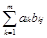.

Denote by Am the block of the matrix A such that Am= (aij)ÎMm´m, i.e. we take the matrix of the first m columns. Then we suppose that we have (Am)tBm = Im, therefore we must have det(Am)¹0. Now, since n>m, we can consider the homogeneous system with respect to the ‘unknowns’ b1n,b2n,…, bmn:

cin=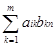= 0 for i= 1,2,…,m.

From which, we obtain that b1n=b2n=…=bmn= 0, since det(Am)¹0. Using this fact on the last equation, on the same unknowns,

cnn=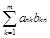=1

we have 0=1, absurd.

We recall some definitions from ,,.

Definition 2.7 Let (G,×) hypergroupoid, is called set of fundamental maps on G, a set of onto maps

Q = { q: G´G®G: (x,y)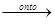z½zÎxy }.

Any subset QsÌQ defines a hope (◦s) on G as follows

x◦sy = { z½z= q(x,y) for some qÎQ }

s £ ×, and QsÌQos, where Qos is the set of fundamental maps with respect to (◦s). A QaÌQ for which every QsÌQa has (◦s) associative (resp. WASS) is called associative (resp. WASS). A hypergroupoid (G,×) is q-WASS if there exists an element qoÎQ which defines an associative operation (◦) in G. Remark that for Hv-groups we have Q¹Æ .

If G is finite, cardG=½G½=n, it is q-WASS with associative qoÎQ. In the set Κ[G] of all formal linear combinations of elements of G with coefficients from a field Κ, we define an operation (+):

(f1+f2)(g)=f1(g)+f2(g),"gÎG,f1,f2ÎΚ[G]

and a hope (*), the convolution,

f1*f2 = { fq: fq(g) =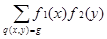, qÎQ }.

(K[G],+,*) is a multiplicative Hv-ring where the inclusion distributivity is valid, which is called hypergroupoid Hv-algebra.

For all qÎQ, gÎG, we have

½Q½£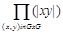, 1 £ |q-1(g)| £ n2-n+1

and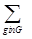|q-1(g)| = n2.

The zero map f(x)=0 is a scalar element in K[G].

In the representation theory several constructions are used, some of them are the following ,,,:

Constructions 2.8 Let (H,×) be Hv-group, then for all (Å) such that xÅyÉ{x,y}, "x,yÎH, the (H,Å,×) is an Hv-ring. These Hv-rings are called associated to (H,×) Hv-rings.

In rep theory of hypergroups, in sense of Marty where the equality is valid, there are three associated hyperrings (H,Å,×) to (H,×). The (Å) is defined respectively, "x,yÎH, by: type a xÅy={x,y}, type b xÅy=β*(x)Èβ*(y), type c xÅy=H.

In the above types the strong associativity and strong or inclusion distributivity, is valid.

Let (H,×) be HV-semigroup and {v1,…,vn}ÇH=Æ, an ordered set, where if vi<vj, when i<j. Extend (×) in Hn=HÈ{v1,v2,…,vn } as follows:

x×vi=vi×x=vi, vi×vj=vj×vi=vj, "i<j and

vi×vi=HÈ{v1,…,vi-1}, "xÎH, iÎ{1,2,…,n}.

Then (Hn,×) is an HV-group (Attach Elements Construction). We have (Hn,×)/β*@Z2 and vn is single.

Some open problems arising on the topic of rep theory of hypergroups, are:

Open Problems.

a. Find standard Hv-rings or Hv-fields to represent all Hv-groups by Hv-matrices.

b. Find reps by Hv-matrices over standard finite Hv-rings analogous to Zn.

c. Using matrices find a generalization of the ordinary multiplication of matrices which it could be used in Hv-rep theory (see the helix-hope ).

d. Find the ‘minimal’ hypermatrices corresponding to the minimal hopes.

e. Find reps of special classes of hypergroups and reduce these to minimal dimensions.

3. Helix-Hopes and Applications

Recall some definitions from ,,:

Definition 3.1Let A=(aij)ÎMm´n be an m´n matrix and s,tÎN be natural numbers such that 1£s£m, 1£t£n. Then we define a characteristic-like map cst: Mm´n®Ms´t by corresponding to the matrix A, the matrix Acst=(aij) where 1£i£s, 1£j£t. We call this map cut-projection of type st. In other words Acst is a matrix obtained from A by cutting the lines, with index greater than s, and columns, with index greater than t.

We can use cut-projections on several types of matrices to define sums and products, however, in this case we have ordinary operations, not multivalued.

In the same attitude we define hopes on any type of matrices:

Definition 3.2 Let A=(aij)ÎMm´n be an m´n matrix and s,tÎN, such that 1£s£m, 1£t£n. We define the mod-like map st from Mm´n to Ms´t by corresponding to A the matrix Ast= (aij) which has as entries the sets

aij = {ai+κs,j+λt½1£i£s, 1£j£t. and κ,λÎN, i+κs£m, j+λt£n}.

Thus we have the map

st: Mm´n®Ms´t: A®Ast = (aij).

We call this multivalued map helix-projection of type st. Thus Ast is a set of s´t-matrices X=(xij) such that xijÎaij,"i,j. Obviously Amn=A. We may define helix-projections on ‘matrices’ of which their entries are sets.

Let A=(aij)ÎMm´n be a matrix and s,tÎN such that 1£s£m, 1£t£n. Then it is clear that we can apply the helix- projection first on the columns and then on the rows, the result is the same if we apply the helix-progection on both, rows and columns. Therefore we have

(Asn)st = (Amt)st = Ast.

Let A=(aij)ÎMm´n be matrix and s,tÎN such that 1£s£m, 1£t£n. Then if Ast is not a set of matrices but one single matrix then we call A cut-helix matrix of type s´t. Thus the matrix A is a helix matrix of type s´t, if Acst= Ast.

Definitions 3.3 (a) Let A=(aij)ÎMm´n and B=(bij)ÎMu´v be matrices and s=min(m,u), t=min(n,u). We define a hope, called helix-addition or helix-sum, as follows:

Å: Mm´n´Mu´v®P(Ms´t):

(A,B)®AÅB=Ast+Bst=(aij)+(bij)Ì Ms´t,

where

(aij)+( bij)= {(cij)= (aij+bij) çaijÎaij and bijÎbij}.

(b) Let A=(aij)ÎMm´n and B=(bij)ÎMu´v be matrices and s=min(n,u). We define a hope, called helix-multiplication or helix-product, as follows:

Ä: Mm´n´Mu´v®P(Mm´v):

(A,B)®AÄB=Ams×Bsv=(aij)×(bij)Ì Mm´v,

where

(aij)×(bij)= {( cij)=(åaitbtj) çaijÎaij and bijÎbij}.

The helix-addition is an external hope since it is defined on different sets and the result is also in different set. The commutativity is valid in the helix-addition. For the helix- multiplication we remark that we have AÄB=Ams×Bsv so we have either Ams=A or Bsv=B, that means that the helix- projection was applied only in one matrix and only in the rows or in the columns. If the appropriate matrices in the helix-sum and in the helix-product are cut-helix, then the result is singleton.

Remark. In Mm´n the addition of matrices is an ordinary operation, therefore we are interested only in the ‘product’. From the fact that the helix-product on non square matrices is defined, the definition of the Lie-bracket is immediate, therefore the helix-Lie Algebra is defined ,, as well. This algebra is an Hv-Lie Algebra where the fundamental relation ε* gives, by a quotient, a Lie algebra, from which a classification is obtained.

In the following we restrict ourselves on the matrices Mm´n where m<n. We have analogous results in the case where m>n and for m=n we have the classical theory. In order to simplify the notation, since we have results on modm, we will use the following notation:

Notation. For given κÎ-{0}, we denote by κ the remainder resulting from its division by m if the remainder is non zero, and κ=m if the remainder is zero. Thus a matrix

A=(aκλ)ÎMm´n, m<n is a cut-helix matrix if aκλ=aκλ, "κ,λÎ-{0}.

Moreover let us denote by Ic=(cκλ) the cut-helix unit matrix which the cut matrix is the unit matrix Im. Therefore, since Im=(δκλ), where δκλ is the Kronecker’s delta, we obtain that, "κ,λ, we have cκλ=δκλ.

Proposition 3.4 For m<n in (Mm´n,Ä) the cut-helix unit matrix Ic=(cκλ), where cκλ=δκλ, is a left scalar unit and a right unit. It is the only one left scalar unit.

Proof. Let A,BÎMm´n then in the helix-multiplication, since m<n, we take helix projection of the matrix A, therefore, the result AÄB is singleton if the matrix A is a cut-helix matrix of type m´m. Moreover, in order to have AÄB=Amm×B=B, the matrix Amm must be the unit matrix. Consequently, Ic=(cκλ), where cκλ=δκλ, "κ,λÎ-{0}, is necessarily the left scalar unit element.

Now we remark that it is not possible to have the same case for the right matrix B, therefore we have only to prove that cut-helix unit matrix Ic is a right unit but it is not a scalar, consequently it is not unique.

Let A=(auv)ÎMm´n and consider the hyperproduct AÄIc. In the entry κλ of this hyperproduct there are sets, for all 1£κ£m, 1£λ£n , of the form

Saκsc = Saκsδ= aκλ' aκλ.

Therefore AÄIc'A, "AÎMm´n.

In the following examples of the helix-hope, we denote Eij any type of matrices which have the ij-entry 1 and in all the other entries we have 0.

Example 3.5  Consider the 2´3 matrices of the following form,

Aκ=E11+κE21+E22+E23, Bκ=κE21+E22+E23, "κÎ.

Then we obtain AκÄAλ={Aκ+λ, Aλ+1,Βκ+λ,Βλ+1}.

Similarly we have ΒκÄAλ={Βκ+λ,Βλ+1}, AκÄΒλλκÄΒλ.

Thus {Aκ,Βλ½κ,λÎ} becomes an Hv-semigroup, not COW because for κ¹λ we have BκÄΒλ=Βλ¹ΒκλÄΒκ, however

(AκÄAλ)Ç(AλÄAκ) = {Aκ+λ,Βκ+λ}¹Æ, "κ,λÎ.

All Βλ are right absorbing and Β1 is a left scalar, because B1ÄAλ=Bλ+1 and B1ÄBλ=Bλ. The A0 is a unit.

Example 3.6 Consider the 2´3 matrices of the forms,

Aκλ=E11+E13+κE21+E22+λE23, "κ,λÎ.

Then we obtain AκλÄAst={Aκ+s,κ+t,Aκ+s,λ+t,Aλ+s,κ+t,Aλ+s,λ+t}.

Moreover AstÄAκλ={Aκ+s,λ+s,Aκ+s,λ+t,Aκ+t,λ+s,Aκ+t,λ+t}, so AκλÄAstÇAstÄAκλ={Aκ+s,λ+t}, thus (Ä) is COW.

The helix multiplication (Ä) is associative.

Example 3.7 Consider all traceless matrices A=(aij)ÎM2´3, in the sence that a11+ a22=0. In this case, the cardinality of the helix-product of any two matrices is 1, or 23, or 26. These correspond to the cases: a11=a13 and a21=a23, or only a11=a13 either only a21=a23, or if there is no restriction, respectively. For the Lie-bracket of two traceless matrices the corresponding cardinalities are up to 1, or 26, or 212, respectively. We remark that, from the definition of the helix-projection, the initial 2´2, block guaranties that in the result there exists at least one traceless matrix.

From this example it is obvious the following:

Theorem 3.8 The Lie-bracket of any two traceless matrices A=(aij), B=(bij)ÎMm´n, m<n, contain at least one traceless matrix.

Last years hyperstructures there is a variety of applications in mathematics and in other sciences. Hyperstructures theory can now be widely applicable in industry and production, too. In several books and papers ,,,,,,, ,,, one can find numerous applications.

The Lie-Santilli theory on isotopies was born in 1970’s to solve Hadronic Mechanics problems. The original theory is reconstructed such as to admit the new matrix as left and right unit. Isofields needed in this theory correspond into the hyperstructures were introduced by Santilli and Vougiouklis in 1996 and they are called e-hyperfields ,,,, . The Hv-fields can give e-hyperfields which can be used in the isotopy theory for applications.

Definitions 3.9 A hyperstructure (H,×) which contain a unique scalar unit e, is called e-hyperstructure, where we assume that "x, there exists an inverse x-1, so eÎx×x-1Çx-1×x. A hyperstructure (F,+,×), where (+) is an operation and (×) is a hope, is called e-hyperfield if the following are valid:

(F,+) is abelian group with the additive unit 0, (×) is WASS,

(×) is weak distributive with respect to (+), 0 is absorbing: 0×x=x×0=0, "xÎF, exist a scalar unit 1, i.e. 1×x=x×1=x, "xÎF,

"xÎF there exists unique inverse x-1, s.t. 1Îx×x-1Çx-1×x.

The elements of an e-hyperfield are called e-hypernumbers. In the case that the relation: 1=x×x-1=x-1×x, is valid, then we say that we have a strong e-hyperfield.

A general construction based on the partial ordering of the Hv-structures:

Construction 3.10 ,, Main e-Construction. Given a group (G,×), where e is the unit, then we define in G, a large number of hopes (Ä) by extended (×), as follows:

xÄy = {xy, g1, g2,…}, "x,yÎG-{e}, and g1, g2,…ÎG-{e}

Then (G,Ä) becomes an Hv-group, in fact is Hb-group which contains the (G,×). The Hv-group (G,Ä) is an e-hypergroup. Moreover, if "x,y such that xy=e, so we have xÄy=xy, then (G,Ä) becomes a strong e-hypergroup.

An application combining hyperstructures and fuzzy theory, is to replace the scale of Likert in questionnaires by the bar of Vougiouklis & Vougiouklis :

Definition 3.11 In every question substitute the Likert scale with ‘the bar’ whose poles are defined with ‘0’ on the left end, and ‘1’ on the right end:

01

The subjects/participants are asked instead of deciding and checking a specific grade on the scale, to cut the bar at any point they feel expresses their answer to the question.

The use of the bar of Vougiouklis & Vougiouklis instead of a scale of Likert has several advantages during both the filling-in and the research processing . The suggested length of the bar, according to the Golden Ratio, is 6.2cm.

References

1. R. Bayon, N. Lygeros, Advanced results in enumeration of hyperstructures, J. Algebra, 320, 2008, 821-835.
2. P. Corsini, V. Leoreanu, Applications of Hypergroup Theory, Kluwer Academic Publ., 2003.
3. P. Corsini, T. Vougiouklis, From groupoids to groups through hypergroups, Rendiconti Mat. VII, 9, 1989, 173-181.
4. B. Davvaz, On Hv-subgroups and anti fuzzy Hv-subgroups, Korean J. Comp. Appl. Math. V.5, N.1, 1998, 181-190.
5. B. Davvaz, Fuzzy Hv-submodules, Fuzzy sets and Systems, 117, 2001, 477-484.
6. B. Davvaz, A brief survey of the theory of Hv-structures, 8th AHA, Greece, Spanidis, 2003, 39-70.
7. B. Davvaz, W. Dudek, T. Vougiouklis, A generalization of n-ary algebraic systems, Communications in Algebra,37, 2009, 1248-1263.
8. B. Davvaz, V. Leoreanu-Fotea, Hyperring Theory and Applications, Int. Academic Press, USA, 2007.
9. B.Davvaz,R.M.Santilli,T.Vougiouklis,Multi-valued Hyper- mathematics for characterization of matter and antimatter systems, J. ComputationalMethodsSciencesEng.13,2013,37–50.
10. B. Davvaz, R.M. Santilli, T. Vougiouklis,Mathematical prediction of Ying’s twin universes, American J. Modern Physics, 4(3), 2015, 5-9.
11. B. Davvaz, S. Vougioukli, T. Vougiouklis, On the multiplicative Hv-rings derived from helix hyperoperations, Util. Math., 84, 2011, 53-63.
12. B. Davvaz, T. Vougiouklis, N-ary hypergroups, Iranian J. of Science & Technology, Transaction A, V.30, N.A2, 2006, 165-174.
13. N.Lygeros,T.Vougiouklis, The LV-hyperstructures, Ratio Math., 25, 2013,59–66.
14. R.M. Santilli, Hadronic Mathematics, Mechanics and Chemistry, Vol. I, II, III, IV and V, Int. Academic Press, USA, 2008.
15. R.M. Santilli, T. Vougiouklis, Isotopies, Genotopies, Hyperstructures and their Appl., Proc. New Frontiers in Hyperstructures and Related Algebras, Hadronic, 1996, 1-48.
16. S. Vougiouklis, Hv-vector spaces from helix hyperoperations, Int. J. Math. Anal. (New Series), 1(2), 2009, 109-120.
17. T. Vougiouklis, Cyclicity in a special class of hypergroups, Acta Un. Car. – Math. Et Ph., V.22, N1, 1981, 3-6.
18. T. Vougiouklis, Representations of hypergroups, Hypergroup algebra, Proc. Convegno: ipergrouppi, altre strutture multivoche appl. Udine, 1985, 59-73.
19. T. Vougiouklis,On affine Kac-Moody Lie algebras, Commentationes Math. Un. Car., V.26, 2, 1985, 387-395
20. T. Vougiouklis, Representations of hypergroups by hyper- matrices, Rivista Mat. Pura Appl., N 2, 1987, 7-19.
21. T. Vougiouklis, Generalization of P-hypergroups, Rend. Circ. Mat. Palermo, S.II, 36, 1987, 114-121.
22. T. Vougiouklis,Groups in hypergroups, Annals of Discrete Math. 37, 1988, 459-468
23. T. Vougiouklis, The fundamental relation in hyperrings. The general hyperfield, Proc. 4thAHA, World Scientific, 1991, 203-211.
24. T. Vougiouklis,Representations of hypergroups by generalized permutations, Algebra Universalis, 29, 1992, 172-183.
25. T. Vougiouklis, Representations of Hv-structures,Proc. Int. Conf. Group Theory 1992, Timisoara,1993, 159-184.
26. T. Vougiouklis,Hyperstructures and their Representations, Monographs in Math., Hadronic Press, 1994.
27. T. Vougiouklis,Some remarks on hyperstructures, Contemp. Math., Amer. Math. Society, 184, 1995, 427-431.
28. T. Vougiouklis, Enlarging Hv-structures, Algebras and Comb., ICAC’97, Hong Kong, Springer-Verlag, 1999, 455-463.
29. T. Vougiouklis,On Hv-rings and Hv-representations, Discrete Math., Elsevier, 208/209, 1999, 615-620.
30. T. Vougiouklis,Finite Hv-structures and their representations, Rend. Seminario Mat. Messina S.II, V.9, 2003, 245-265.
31. T. Vougiouklis, The h/v-structures, J. Discrete Math. Sciences and Cryptography, V.6, 2003, N.2-3, 235-243.
32. T. Vougiouklis,¶-operations and Hv-fields, Acta Math. Sinica, (Engl. Ser.), V.24, N.7, 2008, 1067-1078.
33. T. Vougiouklis, The e-hyperstructures, J. Mahani Math. Research Center, V.1, N.1, 2012, 13-28.
34. T. Vougiouklis, The Lie-hyperalebras and their fundamental relations, Southeast Asian Bull. Math., V.37(4), 2013, 601-614.
35. T. Vougiouklis, Lie-admissible hyperalgebras, Italian J. Pure Applied Math., N.31, 2013,
36. T. Vougiouklis, From Hv-rings to Hv-fields, Int. J. Algebraic Hyperstructures Appl. Vol.1, No.1, 2014, 1-13.
37. T. Vougiouklis, Hypermatrix representations of finite Hv-groups, European J. Combinatorics, V.44 B, 2015, 307-315.
38. T. Vougiouklis,Quiver of hyperstructures for Ying’s twin universes,American J. Modern Physics, 4(1-1), 2015, 30-33.
39. T. Vougiouklis, S. Vougiouklis, The helix hyperoperations, Italian J. Pure Appl. Math., 18, 2005, 197-206.
40. T. Vougiouklis, P. Kambaki-Vougioukli, On the use of the bar, China-USA Business Review, V.10, N.6, 2011, 484-489.

 Contents 1. 2. 3.
Article ToolsAbstractPDF(208K)